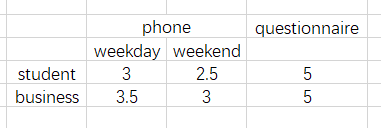# Model building problem

The target of a survey is set as business people and college students, requirements:

1. The total number is 600,and student is not less than 250;
2. the way of survey includes questionnaire and phone, the number is questionnaire should be more than 30%;
3. The students arranged to telephone survey on Saturdays or Sundays should be more than 80%;
4. The business people arranged to phone survey on weekdays should be more than 80 %;

How to arrange the survey to minimize the cost？Here is my sulution:

``````function survey()
N = 600
model = Model(Gurobi.Optimizer);set_silent(model)
@variable(model,x[1:7,1:N],Bin)    # 1 for student
@variable(model,y[1:7,1:N],Bin)    # 1 for questionnaire
@constraint(model,sum(x) >= 250)
@constraint(model,sum(y) >= 0.3N)
``````

But I don;t know how to build the remaining model in code,can someone help me?

I think we’re still missing some necessary information. Based on what you’ve provided, I got this far:

``````using HiGHS
using JuMP
using LinearAlgebra

N = 600
C = [3.0 2.5 5.0; 3.5 3.0 5.0]
model = Model(HiGHS.Optimizer)
n,m = size(C)
@variable(model, R[1:n, 1:m]) # define container for R variables
@constraint(model, sum(R[1, :]) ≥ 250) # total number of students surveyed
@constraint(model, sum(R[:, 3]) ≥ 0.3 * N) # total questionnaires administered
@constraint(model, sum(R) == N) # total number of surveys
@constraint(model, R .≥ 0) # no negative values
@objective(model, Min, dot(C,R)) # minimize cost
optimize!(model)
``````

Questions:

1. Does the total number of surveys have to equal 600 exactly?
2. For constraints 3 and 4, when you say 80%, 80% of what? For example, for constraint 3, should it be 80% of total student surveys administered over the phone, or 80% of total student surveys?

Regardless of the answers for question 2, I’m not sure how to set up those constraints. Assuming that constraint 3 is that at least 80% of student surveys administered by phone should take place on the weekend, I thought about this `@constraint(model, R[1,2] ≥ 0.8 * sum(R[1, 1:2]))` but that won’t work since `sum(R[1, 1:2])` is just 0 before optimizing.

Maybe you can clarify the constraints and then someone else with more JuMP experience can tell you how to go about defining those constraints in the model

1. the total number of surveys have to equal 600 exactly
2. For constraint 3 , it’s 80% of total student surveys administered over the phone,and same as constraint 4.

I also don’t know how to set up the constraints and the objective,so I’m looking for some help.

Got it. What I have should take care of everything except for constraints 3 and 4. I don’t know how to include those, but there are some folks here on the forum that I’m sure will be able to tell you how to do it.

I believe that the 80% constraint refers to telephone surveys, otherwise it would be incompatible with the other 30% constraint on surveys.
If it is correct to write it like this

``````@constraint(model, R[1,2] ≥ 4 * R[1, 1])
@constraint(model, R[2,1] ≥ 4 * R[2, 2])
``````

you get

``````julia> value.(R)
2×3 Matrix{Float64}:
-0.0  420.0  180.0
-0.0   -0.0   -0.0

julia> 420*2.5+180*5
1950.0
``````

I think the issue is that `@constraint(model, R[1,2] ≥ 4 * R[1, 1])` is defined as

-4R_{1,1} + R_{1,2} \ge 0

but I don’t think we want it to be 0, we want that value to be dynamic. Right?

``````@constraint(model, R[1,2] ≥ 4 * R[1, 1]+1)
@constraint(model, R[2,1] ≥ 4 * R[2, 2]+1)

...
Presolve : Reductions: rows 0(-11); columns 0(-6); elements 0(-21) - Reduced to empty
Solving the original LP from the solution after postsolve
Model   status      : Optimal
Objective value     :  1.9510000000e+03
HiGHS run time      :          0.01

julia> value.(R)
2×3 Matrix{Float64}:
-0.0  419.0  180.0
1.0   -0.0   -0.0
``````

I don’t know much about the package (I only used it for one case of interest of mine) and I don’t know how to impose a strict inequality constraint.

PS
Strictly speaking, I think, we should add the constraint of integer solutions, even if we don’t change the result.

PPS
Reasoning “by hand” on the problem, from the cost table, we conclude that we need to use the questionnaire as little as possible (0.3*600=180) and we need to concentrate the interviews on the weekend.
Since there are no links between students and business, everything is done with the students

Interpreting constraints 3 and 4 by column and not by row (i.e. relating to the ratio between students and businesses interviewed on the day of the week)

``````@constraint(model, R[1,2] ≥ 4 * R[2, 2]+1)
@constraint(model, R[2,1] ≥ 4 * R[1, 1]+1)

Objective value     :  1.9510000000e+03
HiGHS run time      :          0.01

julia> value.(R)
2×3 Matrix{Float64}:
-0.0  419.0   -0.0
1.0   -0.0  180.0
``````

Although that’s weird , I can just take it.

@constraint(model, R[1,2] ≥ 4 * R[2, 2]+1)

This is the part I’m struggling with. Is this actually different from `@constraint(model, R[1,2] ≥ 1)`?

I think it is different, may be you can change the data and compare the result.

1 Like

I’d interpret the 80% constraint as this:

``````@constraint(model, R[2,2] >= 0.8 * (R[1,2] + R[2,2]))
``````

in fact ,it’s same as it:

I think it’s better add `+1`,because it’s more than 80%.

I guess it depends on the interpretation of “be more than 80%”